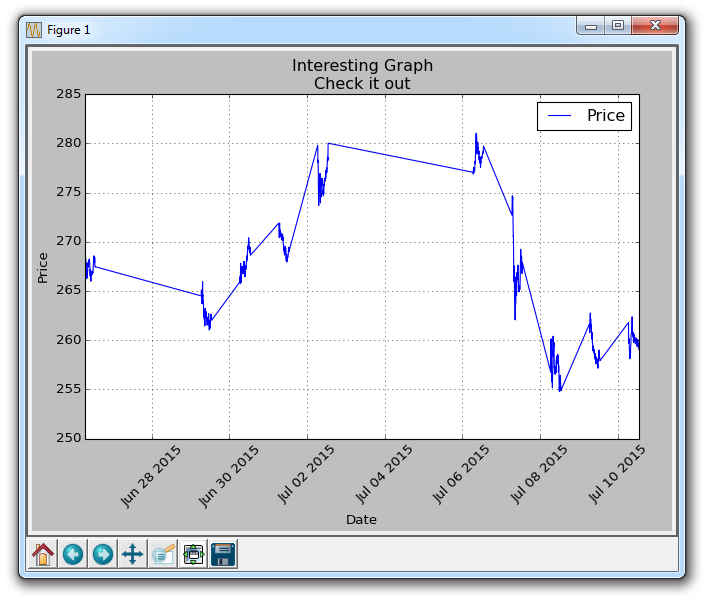# 第十一章 Unix 时间

Unix 时间是 1970 年 1 月 1 日以后的秒数，它是跨程序的标准化时间表示方法。 也就是说，Matplotlib 并不欢迎 unix 时间戳。 这里是你可以使用 Matplotlib 来处理 unix 时间的方式：

``````import matplotlib.pyplot as plt
import numpy as np
import urllib
import datetime as dt
import matplotlib.dates as mdates

def bytespdate2num(fmt, encoding='utf-8'):
strconverter = mdates.strpdate2num(fmt)
def bytesconverter(b):
s = b.decode(encoding)
return strconverter(s)
return bytesconverter

def graph_data(stock):

fig = plt.figure()
ax1 = plt.subplot2grid((1,1), (0,0))

stock_price_url = 'http://chartapi.finance.yahoo.com/instrument/1.0/'+stock+'/chartdata;type=quote;range=10d/csv'
stock_data = []
split_source = source_code.split('\n')
for line in split_source:
split_line = line.split(',')
if len(split_line) == 6:
if 'values' not in line and 'labels' not in line:
stock_data.append(line)

date, closep, highp, lowp, openp, volume = np.loadtxt(stock_data,
delimiter=',',
unpack=True)
dateconv = np.vectorize(dt.datetime.fromtimestamp)
date = dateconv(date)

##    date, closep, highp, lowp, openp, volume = np.loadtxt(stock_data,
##                                                          delimiter=',',
##                                                          unpack=True,
##                                                          converters={0: bytespdate2num('%Y%m%d')})

ax1.plot_date(date, closep,'-', label='Price')
for label in ax1.xaxis.get_ticklabels():
label.set_rotation(45)
ax1.grid(True)#, color='g', linestyle='-', linewidth=5)

plt.xlabel('Date')
plt.ylabel('Price')
plt.title('Interesting Graph\nCheck it out')
plt.legend()
plt.subplots_adjust(left=0.09, bottom=0.20, right=0.94, top=0.90, wspace=0.2, hspace=0)
plt.show()

graph_data('TSLA')
``````

``````dateconv = np.vectorize(dt.datetime.fromtimestamp)
date = dateconv(date)
``````

``````for line in split_source:
split_line = line.split(',')
if len(split_line) == 6:
if 'values' not in line and 'labels' not in line:
stock_data.append(line)
``````